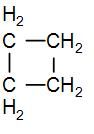QuestionAnswers

# There is no ring strain in cyclohexane, but cyclobutane has an angle strain of ${{9}^{{}^\circ }}{{44}^{'}}$. If $\Delta H_{c}^{\circ }$of cyclohexane per $\left( C{{H}_{2}} \right)$ group is 660 KJ/mol and $\Delta H_{c}^{\circ }$ of cyclobutane is 2744 KJ/mol, what is the ring strain in KJ/mol for cyclobutane?[A] -104[B] 104[C] -2084[D] 2084

Hint: To solve this question, we can use the $\Delta H_{c}^{\circ }$ value of each $\left( C{{H}_{2}} \right)$ groups and the cyclobutane. We can find out the ring strain by subtracting the $\Delta H_{c}^{\circ }$ value of each $\left( C{{H}_{2}} \right)$group present in cyclobutane by the $\Delta H_{c}^{\circ }$ value of cyclobutane. The total number of $\left( C{{H}_{2}} \right)$ groups in cyclobutane is four.

In the given question, we have that the cyclobutane has an angle strain of ${{9}^{{}^\circ }}{{44}^{'}}$. We know that there are four $C{{H}_{2}}$ groups in cyclobutane and we can draw its structure as-As we can see from the above diagram that there are 4 $C{{H}_{2}}$ groups. So to find the ring strain in cyclobutane, we can subtract the standard enthalpy of combustion of each of these $C{{H}_{2}}$ groups from the standard enthalpy of combustion of cyclobutane.
Ring strain = $4\times \Delta H_{c}^{\circ }$ of each $C{{H}_{2}}$ group.-$\Delta H_{c}^{\circ }$of cyclobutane
Therefore, ring strain = $\left( 4\times 660 \right)$KJ/mol - 2274 KJ/mol =140 KJ/mol.
Note: Ring strain is mostly caused due to the $s{{p}^{3}}$ hybridised carbons which are present in the cycloalkanes. For a ring to not be strained the ideal angle is ${{109.5}^{{}^\circ }}$ but due to the $s{{p}^{3}}$ hybridised carbons, the bond angle is not maintained thus causes strain in rings.